# Transformation of Quark Flavors by the Weak Interaction

The decay of hadrons by the weak interaction can be viewed as a process of decay of their constituent quarks. There is a pattern of these quark decays: a quark of charge +2/3 ( u,c,t) is always transformed to a quark of charge -1/3 (d,s,b) and vice versa. This is because the transformation proceeds by the exchange of charged W bosons, which must change the charge by one unit. The general pattern is that the quarks will decay to the most massive quark possible, leading the the pattern

t -> b -> c -> s -> u <-> d

The following table shows the usual pattern of quark transformation and gives examples of some hadron processes to which those quark transformations contribute.

 Quark Process Example Mean Lifetime (s) Up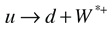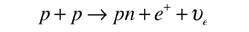... Down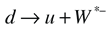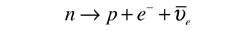900 Strange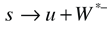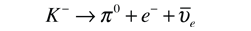1.24 x 10-8 Charm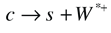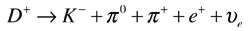1.1 x 10-12 Bottom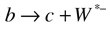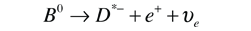1.3 x 10-12 Top... ...

* The W bosons which are indicated as W* are virtual bosons, existing only within the time frame allowed by the uncertainty principle. The positive W* decays to a positron and an electron neutrino, and the negative W* to an electron and antineutrino as can be seen in the example reactions above.

The transformations shown are the most probable for the quarks, but there are other possibilities. The c quark has about 5% probability of decaying into a d quark instead of an s quark.

The most common of the quark transformations are those of the up and down quarks which are the constituents of ordinary matter in the form of protons and neutrons.The decay of the up quark above is important in the proton-proton cycle of nuclear fusion. The decay of the down quark is involved in the decay of the neutron and in beta decay in general.

 Feynman diagrams of transformations
Index

Particle concepts

Reference

Rohlf
Ch. 18, Table 18-1

 HyperPhysics***** Quantum Physics R Nave
Go Back

# Quark Transformations

The modes of quark flavor transformation by the weak interaction are shown in Feynman diagrams. These diagrams are useful in analyzing decay processes to help keep track of what is happening on the quark level. The most common transformations are listed in the quark transformation table. These transformations take place by means of the W vector boson.Index

Particle concepts

Reference

Rohlf
Ch. 18

 HyperPhysics***** Quantum Physics R Nave
Go Back